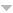# Publications

Found 172 results
[ Author] Title Type Year
Filters: First Letter Of Last Name is R  [Clear All Filters]
R
Minimal presentations of full subsemigroups of $\bold N^2$. Rocky Mountain J. Math.. 2001;31:1417-1422.Edit
The set of solutions of a proportionally modular Diophantine inequality. J. Number Theory. 2008;128:453-467.Edit
Finitely generated commutative monoids Nova Science Publishers, Inc., Commack, NY 1999.Edit
Numerical semigroups. Vol 20 Springer, New York 2009.Edit
Proportionally modular Diophantine inequalities. J. Number Theory. 2003;103:281-294.Edit
On the structure of simplicial affine semigroups. Proc. Roy. Soc. Edinburgh Sect. A. 2000;130:1017-1028.Edit
On Cohen-Macaulay and Gorenstein simplicial affine semigroups. Proc. Edinburgh Math. Soc. (2). 1998;41:517-537.Edit
Arf numerical semigroups. J. Algebra. 2004;276:3-12.Edit
Systems of inequalities and numerical semigroups. J. London Math. Soc. (2). 2002;65:611-623.Edit
Every numerical semigroup is one half of infinitely many symmetric numerical semigroups. Comm. Algebra. 2008;36:2910-2916.Edit
On presentations of commutative monoids. Internat. J. Algebra Comput.. 1999;9:539-553.Edit
$k$-factorized elements in telescopic numerical semigroups. In: Arithmetical properties of commutative rings and monoids. Vol 241. Chapman & Hall/CRC, Boca Raton, FL; 2005. 2. p. 260-271p. (Lect. Notes Pure Appl. Math.; vol 241).Edit
On complete intersection affine semigroups. Comm. Algebra. 1995;23:5395-5412.Edit
Numerical semigroups with maximal embedding dimension. Int. J. Commut. Rings. 2003;2:47-53.Edit
How to check if a finitely generated commutative monoid is a principal ideal commutative monoid. In: Proceedings of the 2000 International Symposium on Symbolic and Algebraic Computation (St. Andrews). ACM, New York; 2000. 2. p. 288-291p. (electronic).Edit
Numerical semigroups with maximal embedding dimension [\refcno 2056070]. In: Focus on commutative rings research. Nova Sci. Publ., New York; 2006. 4. p. 47-53p. Edit
On normal affine semigroups. Linear Algebra Appl.. 1999;286:175-186.Edit
Saturated numerical semigroups. Houston J. Math.. 2004;30:321-330 (electronic).Edit
Presentations of finitely generated submonoids of finitely generated commutative monoids. Internat. J. Algebra Comput.. 2002;12:659-670.Edit
Presentations for subsemigroups of finitely generated commutative semigroups. Israel J. Math.. 1999;113:269-283.Edit
Numerical semigroups with a monotonic Apéry set. Czechoslovak Math. J.. 2005;55(130):755-772.Edit
Nonnegative elements of subgroups of $\bf Z^n$. Linear Algebra Appl.. 1998;270:351-357.Edit
Constructing almost symmetric numerical semigroups from irreducible numerical semigroups. Comm. Algebra. 2014;42:1362-1367.Edit
Atomic commutative monoids and their elasticity. Semigroup Forum. 2004;68:64-86.Edit